# Tests of Adequacy - Index Numbers, Business Mathematics and Statistics - Business Mathematics and Statistics - B Com

So far we have discussed various formulae for construction of weighted & unweighted index numbers.
However the problem still remains of selecting an appropriate method for the construction of an index number in a given situation. The following tests can be applied to find out the adequacy of an index number.

(1) Unit Test
(2) Time Reversal Test
(3) Factor Reversal Test
(4) Circular Test

1. Unit Test - This test requires that the index number formulae should be independent of the units in which prices or quantities of various commodities are quoted. For example in a group of commodities, while the price of wheat might be in kgs., that of vegetable oil may be quoted in per liter & toilet soap may be per unit.
Except for the simple (unweighted) aggregative index, all other formulae discussed above satisfy this test.

2. Time Reversal Test - The time reversal test is used to test whether a given method will work both backwards & forwards with respect to time. The test is that the formula should give the same ratio between one point of comparison & another no matter which of the two is taken as base.
The time reversal test may be stated more precisely as follows— If the time subscripts of a price (or quantity) index number formula be interchanged, the resulting price (or quantity) formula should be reciprocal of the original formula. i.e. if p0 represents price of year 2011 and p1 represents price at year 2012 i.e.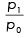should be equal to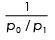symbolically, the following relation should be satisfied p01 x p10 = 1, Omitting the factor 100 from both the indices.
Where P01 is index for current year ‘1’ based on base year ‘0’ pl0 is index for year ‘0’ based on year ‘1’.

The methods which satisfy the following test are:-

(1) Simple aggregate index
(2) Simple geometric mean of price relative
(3) Weighted geometric mean of price relative with fixed weights
(4) Kelly’s fixed weight formula
(5) Fisher’s ideal formula
(6) Marshall-Edgeworth formula

This test is not satisfied by Laspeyres’ method & the Paasche’s method as can be seen from below—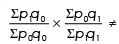1 (Laspeyres'Method)

Similarly when Paasche method is used—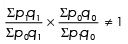On other hand applying Fisher’s formula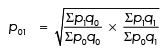(Omitting the factor 100)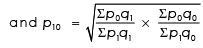(Omitting the factor 100)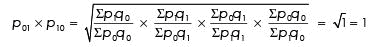Hence the test is satisfied.

3. Factor Reversal Test - An Index number formula satiesfies this test if the product of the Price Index and the Quantity Index gives the True value ratio, omitting the factor 100 from each index. This test is satisfied if the change in the price multiplied by the change in quantity is equal to the change in the value.

Speaking precisely if p and q factors in a price (or quantity) index formula be interchanged, so that a quantity (or price) index formula is obtained the product of the two indices should give the true value ratio.

Symbolically,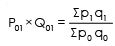= The True Value Ratio = TVR

Consider the Laspeyres formula of price index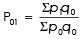Consider the quantity index by interchange p with q & q with p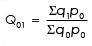Now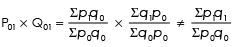This test is not met.
This test is only met by Fisher’s ideal index. No other index number satisfies this test:

Proof :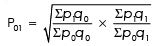Changing p to q and q to p, we get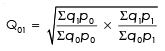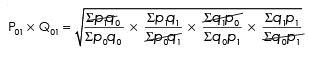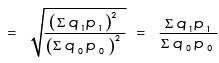= True Value Ratio

4. Circular Test - Circular test is an extension of time reversal test for more than two periods & is based on shiftability of the base period. For example, if an index is constructed for the year 2012 with the base of 2011 & another index for 2011 with the base of 2010. Then it should be possible for us to directly get an index for the year 2012 with the base of 2010. If the index calculated directly does not give an inconsistent value, the circular test is said to be satisfied.

This test is satisfied if— P01 x P12 x P20= 1.

This test is satisfied only by the following index Nos. formulas—

(1) Simple aggregative index
(2) Simple geometric mean of price relatives
(3) Kelly’s fixed base method When the test is applied to simple aggregative method—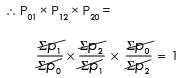Hence, the simple aggregative formula satisfies circular test Similarly when it is applied to fixed weight Kelly’s method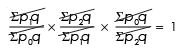This test is not satisfied by Fishers ideal index.

Example 7 : Compute Fisher’s Ideal Index and show that it satisfies time Reversal Test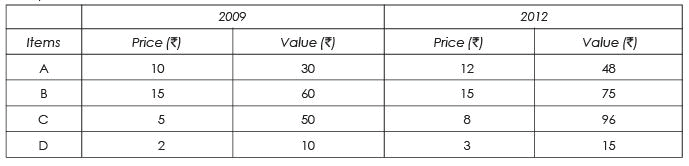Solution :
As in this problem value of each item is given we have to find out quantity by dividing the value by the price. Symbolically : Value = Price x Quantity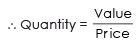Table : Calculation of Fisher’s Ideal Index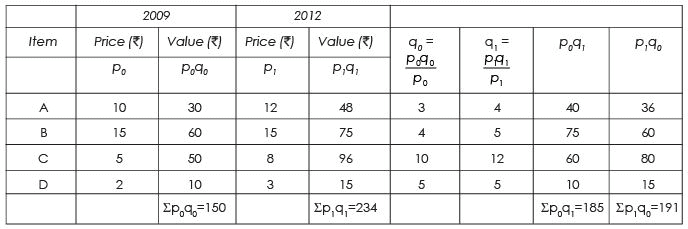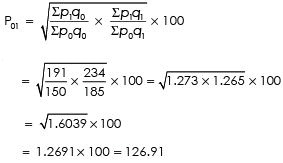Time reversal test is satisfied when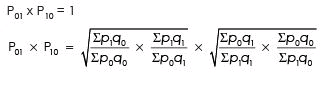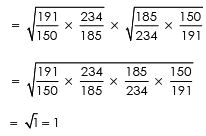Since P01 x P10 = 1 hence Fisher’s ideal index satisfies time Reversal Test.

The document Tests of Adequacy - Index Numbers, Business Mathematics and Statistics | Business Mathematics and Statistics - B Com is a part of the B Com Course Business Mathematics and Statistics.
All you need of B Com at this link: B Com

115 videos|142 docs

## FAQs on Tests of Adequacy - Index Numbers, Business Mathematics and Statistics - Business Mathematics and Statistics - B Com

 1. What are tests of adequacy in the context of index numbers?Ans. Tests of adequacy refer to the statistical methods used to evaluate the suitability of an index number for measuring changes in a particular variable over time. These tests aim to ensure that the index number accurately reflects the changes in the variable it measures and that it is a reliable indicator of the economic trends it represents.
 2. What are some common tests of adequacy used in index number analysis?Ans. Some common tests of adequacy used in index number analysis include the time-reversal test, factor-reversal test, circular test, and mean-relative divergence test. These tests evaluate the index number's consistency, stability, and directionality, and help to identify any bias or errors in the index number construction.
 3. How are tests of adequacy useful in business and economics?Ans. Tests of adequacy are useful in business and economics as they help to ensure the accuracy and reliability of index numbers as a tool for measuring economic trends. By using these tests, analysts can identify and correct any issues with the construction of the index number, which can help to improve the accuracy of economic forecasts and inform policy decisions.
 4. What are the limitations of tests of adequacy in index number analysis?Ans. Although tests of adequacy are useful in evaluating index numbers, they have some limitations. For example, these tests assume that the data is homogenous and that there are no outliers or extreme values that could affect the index number's accuracy. Additionally, these tests do not provide any information about the causes of any inconsistencies or biases in the index number construction.
 5. How can businesses and analysts use index numbers to make informed decisions?Ans. Businesses and analysts can use index numbers to make informed decisions by interpreting the trends and changes in the index number over time. For example, if an index number measuring commodity prices shows an increase over time, this could indicate inflationary pressures in the economy, which could inform decisions about pricing, investment, and risk management. Additionally, businesses and analysts can use index numbers to compare economic trends across different regions and industries, which can help to identify opportunities for growth and development.

115 videos|142 docsExplore Courses for B Com examSignup to see your scores go up within 7 days! Learn & Practice with 1000+ FREE Notes, Videos & Tests.
10M+ students study on EduRev
Track your progress, build streaks, highlight & save important lessons and more!
Related Searches

,

,

,

,

,

,

,

,

,

,

,

,

,

,

,

,

,

,

,

,

,

,

,

,

;